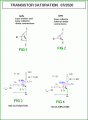# I really can't find a solution for this, need to find how many Ohms is the resistor

Joined Jun 30, 2020
6
Okay so I have a project due tomorrow and I can't get it right, I need to find how many Ohms is the resistor Rb, the link is a screenshot to the task UBX is Uin. https://prnt.sc/t92gbh

#### AlbertHall

Joined Jun 4, 2014
12,202
Are you trying to get a particular collector voltage?

Joined Jun 30, 2020
6
It's actually my bad, I'm looking for the highest value of Rb, which will allow the transistor to be in state of saturation.

Joined Jun 30, 2020
6
I built this circuit in Proteus 8, but I'm not sure if it's right. We're all quite rusty, since nobody tried to explain anything to us during the COVID situation, so we're left with a bunch of PDF files and a couple of problems to solve..

#### MrChips

Joined Oct 2, 2009
28,151
You know the maximum collector current is 14mA.
Use β = 10 to calculate the base current for transistor in saturation.

Joined Jun 30, 2020
6
I am not quite sure which formula am I supposed to use, everything is so different, compared to the bulgarian PDF file, I found this formula in google, but which current is Ic?

#### MrChips

Joined Oct 2, 2009
28,151
IC = β x IB

Is this homework?

Joined Jun 30, 2020
6
It's like homework, but it'll be a big part of the final grade. But I can't call it a homework, since nobody has taught us anything about transistors and how do they work.

#### MrChips

Joined Oct 2, 2009
28,151
The maximum collector current Ic is Vcc / Rc = 14V / 1kΩ = 14mA.

Use β = 10 for worst case scenario.
Calculate IB = IC / β

RB = (Vin - VBE) / IB

Assume VBE = 0.7V

Joined Jun 30, 2020
6
Okay, I did it, thanks a lot for the help, I'll send him the solved problem in a bit. Have a great day!

#### BobaMosfet

Joined Jul 1, 2009
2,069
Okay so I have a project due tomorrow and I can't get it right, I need to find how many Ohms is the resistor Rb, the link is a screenshot to the task UBX is Uin. https://prnt.sc/t92gbh
Title: Understanding Basic Electronics, 1st Ed.
Publisher: The American Radio Relay League
ISBN: 0-87259-398-3

Read the section on BJT transistors, alpha and beta gain calculations.

#### Audioguru again

Joined Oct 21, 2019
5,585
The Bulgarian file is wrong using the Beta of the transistor to calculate the base resistor when the transistor is a saturated switch. The datasheet of your old obsolete transistor and nearly every other transistor shows that beta is used when the transistor has a collector voltage of 10V so it is not saturated. The datasheet shows a base current that is 1/10th the collector current for the transistor to be saturated. Maybe your teacher also does not know and wants you to learn it wrong?

#### MrAl

Joined Jun 17, 2014
9,781
Okay so I have a project due tomorrow and I can't get it right, I need to find how many Ohms is the resistor Rb, the link is a screenshot to the task UBX is Uin. https://prnt.sc/t92gbh
Hello there,

Here is a basic rundown of how saturation of a bipolar transistor is characterized.

(See attached drawing)
In FIG 1 we see the NPN transistor internal diodes, the BE diode and the BC diode.
In FIG 2 we see just the BC diode and that is the diode we want to concentrate on.

When the BC diode first becomes forward biased that is when the transistor starts to enter saturation. If we choose 0.7v nominal for the BE diode and 0.5v for the point where the BC diode just starts to conduct, then in FIG 3 the transistor is not yet into saturation because the BC diode only has 0.49v across it and it should have 0.50v in order for the transistor to begin to enter saturation.
In FIG 4 however, we see the BC diode does have 0.50v across it so it begins to conduct in the forward direction and that means the transistor is just starting to enter saturation.

You might note that in FIG 3 the collector emitter voltage is slightly higher than in FIG 4.

Now since the transistor enters sat when the BC diode has 0.50v across it and so starts to conduct in the forward direction, that means the the collector voltage for this particular transistor must be 0.20 volts. Given the applied voltage to the base resistor is 8v and the BE diode normal forward bias voltage is 0.7v, that leaves 7.3v across the base resistor Rb.
With 7.3v across Rb the current in Rb is Ib=7.3/Rb, and with a Beta of 55 that means the collector current is Ic=55*Ib=55*7.3/Rb. The voltage DROP across Rc is therefore:
vRc=Ic*Rc=Rc*401.5/Rb and that puts the collector voltage at:
Vc=Vcc-Rc*401.5/Rb
and since Vcc=14v and we need Vc to be 0.20v in order to first enter saturation, we can form the equation:
0.20=14-Rc*401.5/Rb
and since Rc=1000 we get:
0.20=14-1000*401.5/Rb
Here we are left with just one variable, the base resistor Rb and you should be able to calculate that from here.Last edited: